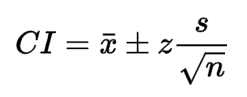Request a Tool

Confidence Interval Calculator

Confidence Interval Calculator use to calculate confidence interval by mean, sd and also by enter raw data.

Output

Confidence Interval
0

Formula• CI = confidence interval
• x = sample mean
• z = confidence level value
• s = sample standard deviation
• n = sample size

Defination / Uses

If you don't know your population mean () but know the standard deviation (), you can use the formula x z* / (n) to calculate a confidence interval for the population mean.

Output

Confidence Interval
0

Formula• CI = confidence interval
• x = sample mean
• z = confidence level value
• s = sample standard deviation
• n = sample size

Defination / Uses

If you don't know your population mean () but know the standard deviation (), you can use the formula x z* / (n) to calculate a confidence interval for the population mean.

"A confidence interval is the range of values, calculated from sample statistics, that is likely to include the value of an unknown population parameter," according to the definition. Consider a brick maker who is concerned about whether the volume of bricks he produces meets specifications. He determined that the average mass of a sample of 100 bricks was 3 kg. He also discovered that the 95 percent confidence interval is between 2.85 and 3.15 kg. It means he can be 95 percent certain that the average mass of all the bricks he makes will be between 2.85 and 3.15 kilogrammes. Of course, you don't need to be 95% certain all of the time.